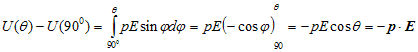# Electric Potential

## 7. Potential due to an electric dipole

• We already know that electric dipole is an arrangement which consists of two equal and opposite charges +q and -q separated by a small distance 2a.
• Electric dipole moment is represented by a vector p of magnitude 2qa and this vector points in direction from -q to +q.
• To find electric potential due to a dipole consider charge -q is placed at point P and charge +q is placed at point Q as shown below in the figure.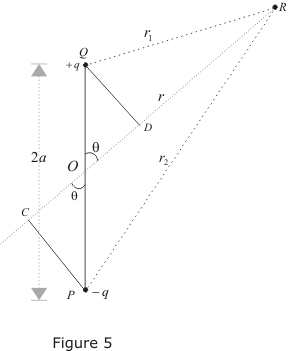• Since electric potential obeys superposition principle so potential due to electric dipole as a whole would be sum of potential due to both the charges +q and -q. Thus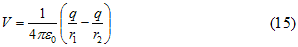where r1 and r2 respectively are distance of charge +q and -q from point R.
• Now draw line PC perpandicular to RO and line QD perpandicular to RO as shown in figure. From triangle POC
cosθ=OC/OP = OC/a
therefore OC=acosθ similarly OD=acosθ
Now ,
r1 = QR≅RD = OR-OD = r-acosθ
r2 = PR≅RC = OR+OC = r+acosθ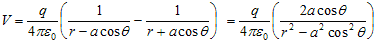since magnitude of dipole is
|p| = 2qa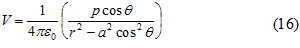• If we consider the case where r>>a then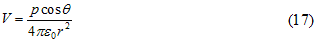again since pcosθ= p· where, is the unit vector along the vector OR then electric potential of dipole is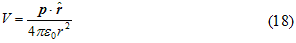for r>>a
• From above equation we can see that potential due to electric dipole is inversly proportional to r2 not ad 1/r which is the case for potential due to single charge.
• Potential due to electric dipole does not only depends on r but also depends on angle between position vector r and dipole moment p.

## 8. Work done in rotating an electric dipole in an electric field

• Consider a dipole placed in a uniform electric field and it is in equilibrium position. If we rotate this dipole from its equllibrium position , work has to be done.
• Suppose electric dipole of moment p is rotated in uniform electric field E through an angle θ from its equilibrium position. Due to this rotation couple acting on dipole changes.
• If at any instant dipole makes an angle φ with uniform electric field then torque acting on dipole is
Γ=pEsinφ                                                    (19)
again work done in rotating this dipole through an infitesimaly small angle dφ is
dW=torque x angular displacement
=pEsinφdφ
• Total work done in rotating the dipole through an angle θfrom its equilibrium position is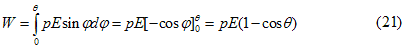This is the required formula for work done in rotating an electric dipole placed in uniform electric field through an angle θ from its equilibrium position.

## 9.Potential energy of dipole placed in uniform electric field

• Again consider equation 20 which gives the work done in rotating electric dipole through an infinetesimly small angle dφ is
dW=pEsinφdφ
which is equal to the change in potential energy of the system
dW=dU=pEsinφdφ                                                    (22)
• If angle dφ is changed from 900 to θ then in potential energy would be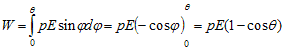• We have choosen the value of φgoing from π/2 to θ because at π/2 we can take potential energy to be zero (axis of dipole is perpandicular to the field). Thus U(900)=0 and above equation becomes